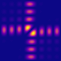QPMS Electromagnetic multiple scattering library and toolkit.
groups.h
Go to the documentation of this file.
1
24 #ifndef QPMS_GROUPS_H
25 #define QPMS_GROUPS_H
26
27 #include "qpms_types.h"
28 #include <assert.h>
29
32  int dim;
33  char *name;
35
38  complex double *m;
39 };
40
42
53 typedef struct qpms_finite_group_t {
54  char *name;
60  int ngens;
62  char **elemlabels;
64  struct qpms_irot3_t *rep3d;
68
71  qpms_gmi_t a, qpms_gmi_t b) {
72  assert(a < G->order);
73  assert(b < G->order);
74  return G->mt[G->order * a + b];
75 }
76
79  qpms_gmi_t a) {
80  assert(a < G->order);
81  return G->invi[a];
82 }
83
84 static inline _Bool qpms_iri_is_valid(const qpms_finite_group_t *G, qpms_iri_t iri) {
85  return (iri > G->nirreps || iri < 0) ? 0 : 1;
86 }
87
88
89
92
95
96 #endif // QPMS_GROUPS_H
static qpms_gmi_t qpms_finite_group_mul(const qpms_finite_group_t *G, qpms_gmi_t a, qpms_gmi_t b)
Group multiplication.
Definition: groups.h:70
const qpms_finite_group_t QPMS_FINITE_GROUP_TRIVIAL_G
Trivial group, with one (reduntant) generator.
Definition: trivialgroup.c:9
const qpms_finite_group_t QPMS_FINITE_GROUP_TRIVIAL
Trivial group.
Definition: trivialgroup.c:40
static qpms_gmi_t qpms_finite_group_inv(const qpms_finite_group_t *G, qpms_gmi_t a)
Group element inversion.
Definition: groups.h:78
struct qpms_finite_group_t qpms_finite_group_t
A point group with its irreducible representations and some metadata.
qpms_iri_t qpms_finite_group_find_irrep_by_name(qpms_finite_group_t *G, char *name)
NOT IMPLEMENTED Get irrep index by name.
Common qpms types.
int qpms_gmi_t
Definition: qpms_types.h:320
int qpms_iri_t
Definition: qpms_types.h:323
const char * qpms_permutation_t
Permutation representation of a group element.
Definition: qpms_types.h:330
To be used only in qpms_finite_group_t.
Definition: groups.h:31
complex double * m
Irrep matrix data.
Definition: groups.h:38
char * name
Definition: groups.h:33
int dim
Irrep dimension.
Definition: groups.h:32
A point group with its irreducible representations and some metadata.
Definition: groups.h:53
qpms_gmi_t order
Group order (number of elements)
Definition: groups.h:55
int ngens
Number of the generators in gens;.
Definition: groups.h:60
char ** elemlabels
Optional human readable labels for the group elements.
Definition: groups.h:62
qpms_iri_t nirreps
How many irreps does the group have.
Definition: groups.h:65
qpms_gmi_t * invi
Group elem inverse indices.
Definition: groups.h:58
qpms_gmi_t idi
Identity element index.
Definition: groups.h:56
struct qpms_irot3_t * rep3d
The quaternion representation of a 3D point group (if applicable).
Definition: groups.h:64
qpms_gmi_t * mt
Group multiplication table. If c = a*b, then ic = mt[order * ia + ib].
Definition: groups.h:57
int permrep_nelem
Number of the elements over which the permutation representation acts.
Definition: groups.h:63
qpms_permutation_t * permrep
Permutation representations of the elements.
Definition: groups.h:61
struct qpms_finite_group_irrep_t * irreps
Irreducible representations of the group.
Definition: groups.h:66
qpms_gmi_t * gens
A canonical set of group generators.
Definition: groups.h:59
3D improper rotations represented as a quaternion and a sign of the determinant.
Definition: qpms_types.h:275